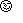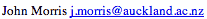Data Structures and Algorithms 10 Graphs

### 10.1 Minimum Spanning Trees

#### Greedy Algorithms

Many algorithms can be formulated as a finite series of guesses, eg in the Travelling Salesman Problem, we try (guess) each possible tour in turn and determine its cost. When we have tried them all, we know which one is the optimum (least cost) one. However, we must try them all before we can be certain that we know which is the optimum one, leading to an O(n!) algorithm.

Intuitive strategies, such as building up the salesman's tour by adding the city which is closest to the current city, can readily be shown to produce sub-optimal tours. As another example, an experienced chess player will not take an opponent's pawn with his queen - because that move produced the maximal gain, the capture of a piece - if his opponent is guarding that pawn with another pawn. In such games, you must look at all the moves ahead to ensure that the one you choose is in fact the optimal one. All chess players know that short-sighted strategies are good recipes for disaster!

There is a class of algorithms, the greedy algorithms, in which we can find a solution by using only knowledge available at the time the next choice (or guess) must be made. The problem of finding the Minimum Spanning Tree is a good example of this class.

#### The Minimum Spanning Tree Problem

Suppose we have a group of islands that we wish to link with bridges so that it is possible to travel from one island to any other in the group. Further suppose that (as usual) our government wishes to spend the absolute minimum amount on this project (because other factors like the cost of using, maintaining, etc, these bridges will probably be the responsibility of some future government). The engineers are able to produce a cost for a bridge linking each possible pair of islands. The set of bridges which will enable one to travel from any island to any other at minimum capital cost to the government is the minimum spanning tree.

We will need some definitions first:

#### Graphs

A graph is a set of vertices and edges which connect them. We write:

G = (V,E)

where V is the set of vertices and the set of edges,

E = { (vi,vj) }
where vi and vj are in V.

#### Paths

A path, p, of length, k, through a graph is a sequence of connected vertices:

p = <v0,v1,...,vk>
where, for all i in (0,k-1:

(vi,vi+1) is in E.

#### Cycles

A graph contains no cycles if there is no path of non-zero length through the graph, p = <v0,v1,...,vk> such that v0 = vk.

#### Spanning Trees

A spanning tree of a graph, G, is a set of |V|-1 edges that connect all vertices of the graph.

#### Minimum Spanning Tree

In general, it is possible to construct multiple spanning trees for a graph, G. If a cost, cij, is associated with each edge, eij = (vi,vj), then the minimum spanning tree is the set of edges, Espan, forming a spanning tree, such that:

C = sum( cij | all eij in Espan )

is a minimum.

#### Kruskal's Algorithm

This algorithm creates a forest of trees. Initially the forest consists of n single node trees (and no edges). At each step, we add one (the cheapest one) edge so that it joins two trees together. If it were to form a cycle, it would simply link two nodes that were already part of a single connected tree, so that this edge would not be needed.

The basic algorithm looks like this:

```Forest MinimumSpanningTree( Graph g, int n, double **costs ) {
Forest T;
Queue q;
Edge e;
T = ConsForest( g );
q = ConsEdgeQueue( g, costs );
for(i=0;i<(n-1);i++) {
do {
e = ExtractCheapestEdge( q );
} while ( Cycle( e, T ) );
}
return T;
}
```
The steps are:
1. Construct a forest - with each node in a separate tree.
2. Place the edges in a priority queue.
3. Until we've added n-1 edges,
1. Continue extracting the cheapest edge from the queue,
until we find one that does not form a cycle,
2. Add it to the forest. Adding it to the forest will join two trees together.
Every step joins two trees in the forest together, so that, at the end, only one tree will remain in T.

We can use a heap for the priority queue. The trick here is to detect cycles. For this, we need a union-find structure.

#### Union-find structure

To understand the union-find structure, we need to look at a partition of a set.

#### Partitions

A partitions is a set of sets of elements of a set.
• Every element of the set belong to one of the sets in the partition.
• No element of the set belong to more than one of the sub-sets.
or
• Every element of a set belongs to one and only one of the sets of a partition.

The forest of trees is a partition of the original set of nodes. Initially all the sub-sets have exactly one node in them. As the algorithm progresses, we form a union of two of the trees (sub-sets), until eventually the partition has only one sub-set containing all the nodes.

A partition of a set may be thought of as a set of equivalence classes. Each sub-set of the partition contains a set of equivalent elements (the nodes connected as one of the trees of the forest). This notion is the key to the cycle detection algorithm. For each sub-set, we denote one element as the representative of that sub-set or equivalence class. Each element in the sub-set is, somehow, equivalent and represented by the nominated representative.

As we add elements to a tree, we arrange that all the elements point to their representative. As we form a union of two sets, we simply set the representative of one of the sets to point to any element of the other set.

So the test for a cycle reduces to: for the two nodes at the ends of the candidate edge, find their representatives. If the two representatives are the same, the two nodes are already in a connected tree and adding this edge would form a cycle. The search for the representative simply follows a chain of links.

Each node will need a representative pointer. Initially, each node is its own representative, so the pointer is set to NULL. As the initial pairs of nodes are joined to form a tree, the representative pointer of one of the nodes is made to point to the other, which becomes the representative of the tree. As trees are joined, the representative pointer of the representative of one of them is set to point to any element of the other. (Obviously, representative searches will be somewhat faster if one of the representatives is made to point directly to the other.)

 Equivalence classes also play an important role in the verification of software.

Select diagrams of Kruskal's algorithm in operation.

#### Greedy operation

At no stage did we try to look ahead more than one edge - we simply chose the best one at any stage. Naturally, in some situations, this myopic view would lead to disaster! The simplistic approach often makes it difficult to prove that a greedy algorithm leads to the optimal solution. proof by contradiction is a common proof technique used: we demonstrate that if we didn't make the greedy choice now, a non-optimal solution would result. Proving the MST algorithm
is, happily, one of the simpler proofs by contradiction!

#### Data structures for graphs

You should note that we have discussed graphs in an abstract way: specifying that they contain nodes and edges and using operations like AddEdge, Cycle, etc. This enables us to define an abstract data type without considering implementation details, such as how we will store the attributes of a graph! This means that a complete solution to, for example, the MST problem can be specified before we've even decided how to store the graph in the computer. However, representation issues can't be deferred forever, so we need to examine ways of representing graphs in a machine. As before, the performance of the algorithm will be determined by the data structure chosen.

### Key terms

Greedy algorithms
Algorithms which solve a problem by making the next step based on local knowledge alone - without looking ahead to determine whether the next step is the optimal one.
Equivalence Classes
The set of equivalence classes of a set is a partition of a set such that all the elements in each subset (or equivalence class) is related to every other element in the subset by an equivalence relation.
Union Find Structure
A structure which enables us to determine whether two sets are in fact the same set or not.
Kruskal's Algorithm
One of the two algorithms commonly used for finding a minimum spanning tree - the other is Prim's algorithm.

©, 1998